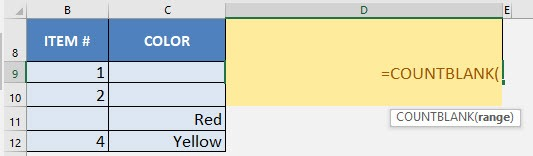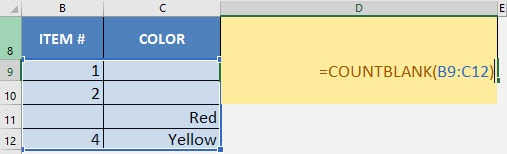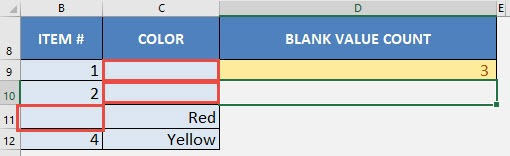What does it do?

Counts the number of cells that are blank

Formula breakdown:

=COUNTBLANK(range)

What it means:

=COUNTBLANK(range of cells to check)

Do you have a scenario where you want to count the number of cells that are blank in your Excel data?

If you are auditing your data and want to make sure that a blank cell is actually blank (and doesn’t contain an invisible character), then this formula is for you.

There is a simple way to count blank cells using Excel’s COUNTBLANK formula!

I explain how you can do this below:

STEP 1: We need to enter the COUNTBLANK function in a blank cell:

## =COUNTBLANK(STEP 2: The COUNTBLANK arguments:

## range

What is the range of values that you want to check how many are blank?

## =COUNTBLANK(B9:C12)You now have your count of values that are blank! There are 3 blank values!CountBlank Formula in Excel